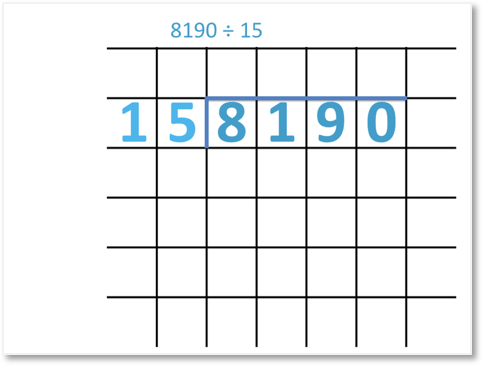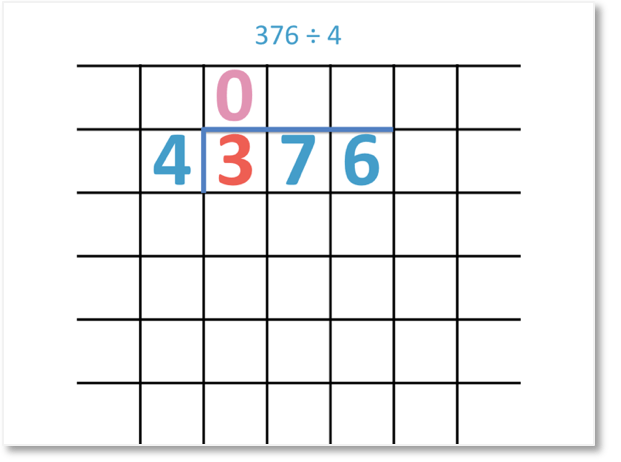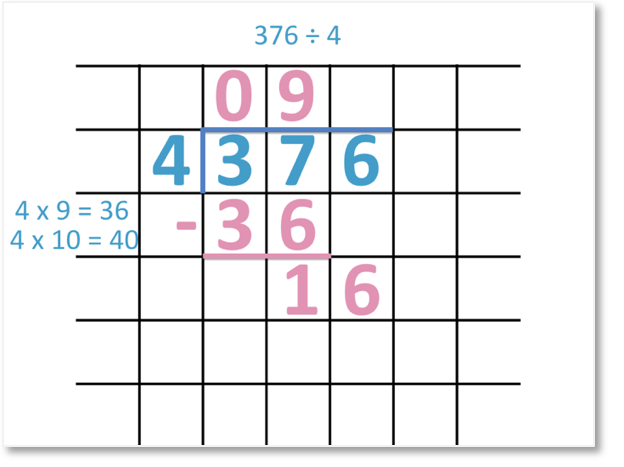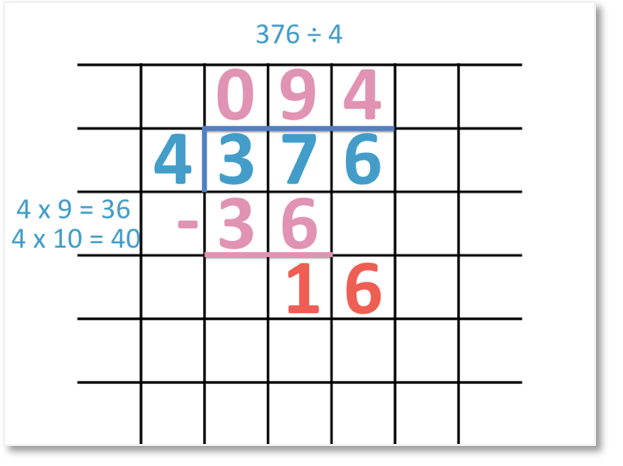# How to Do Long Division

How to do Long Division• Write the number being divided to the right of the number you are dividing by, separated by a dividing line.
• We are dividing 376 by 4.
• We divide each digit separately from left to right.
• 3 ÷ 4 = 0 remainder 4.
• We write the 0 above the 3 and carry the remainder over to the next digit along.
• We now divide 37 by 4.
• 4 × 9 = 36 and 4 × 10 = 40. Therefore 4 divides into 37 9 times.
• We write 9 above and subtract 36 from 37 to get a remainder of 1.
• We bring down the remaining digit of 6 next to the 1 to make 16.
• 16 ÷ 4 = 4 and so, we write 4 above.
• 376 ÷ 4 = 94.
Divide each digit from left to right.

Subtract the largest multiple that goes into each number to find the remainder.• Here we divide 8190 by the two digit number of 15.
• 8 ÷ 15 = 0 remainder 8. We write a 0 above.
• The remainder of 8 is carried over to the next digit to make 81.
• We can list the multiples of 15 to help us do larger divisions.
• 5 × 15 = 75 and so 15 divides into 81 five times. We write 5 above.
• We subtract 75 from 81 to see there is a remainder of 6.
• We write the next digit of 9 next to this remainder to make 69.
• 4 × 15 = 60 and so 15 divides into 69 four times. We write 4 above.
• We subtract 60 from 69 to get a remainder of 9.
• We write the next digit of 0 next to this remainder to make 90.
• 90 ÷ 15 = 6 and so, we write 6 above.
• There are no more digits and remainders, therefore we have our final answer. 8190 ÷ 15 = 546.#### Long Division by Two Digits# Long Division

## What is the Long Division Method

Long division is a method used for dividing by larger numbers. Long division is different from short division in that more working out is shown at each stage in order to calculate the remainders.

The long division method is used when dividing by a number that has two or more digits.

The reason we use long division is to provide a structure for dividing by larger numbers. Long division involves writing out the calculations involved in finding a remainder. By showing this level of working out, there is less chance of making a mistake.

## How to do Long Division

To do long division, follow these steps:

1. Make a list of the multiples of the number being divided by.
2. Use this list to divide the first digit by this number.
3. Find the greatest number of times that the number divides into the digit and write this above.
4. Subtract the largest multiple of the number from the digit being divided to find the remainder.
5. Write the next digit of the number being divided alongside this remainder.
6. Repeat steps 3 to 5 until there are no more remainders.

Here is an example of dividing a number using long division step-by-step.

In this question we have 8190 ÷ 15.

To set out long division, write the number being divided by to the left of the number being divided. Draw a line to separate the numbers which passes above the number being divided.The first step is to make a list of the first few multiples of the number being divided by.

The first few multiples of 15 are: 15, 30, 45, 60, 75, 90.

THe next step is to divide the first digit. 15 is larger than 8 and so, we simply write a zero above the 8 because 15 divides into 8 zero times.We now carry the 8 over as a remainder to make 81. We use the list of multiples to decide how many times 15 divides into 81.

5 × 15 = 75 and 6 × 15 = 90. Therefore, 15 divides into 81 five times. We write a 5 above the line.

The largest multiple of 15 that is less than 81 is 75. The next step is to find the remainder by subtracting 75 from 81.81 – 75 = 6 and so, the remainder is 6.

The next step is to bring the next digit of the number down alongside this remainder. The next digit of 8190 to consider is the 9. We write the 9 next to the remainder of 6 to make 69.We now divide 69 by 15.

4 × 15 = 60 and 5 × 15 = 75. Therefore 15 divides into 69 four times. We write a 4 above the line.

The next step is to subtract the largest multiple of 15 that is less than 69. We subtract 60 from 69 to find the remainder.

69 – 60 = 9 and so, the remainder is 9.The next step is to bring the next digit of 8190 down next to the remainder. The next digit to consider is the 0 and so, we bring this 0 down next to the 9 to make 90.The next step is to divide 90 by 15.

6 × 15 = 90 and so, 90 divided by 15 equals 6. We write a 6 above the line.

We know that the long division method is complete when there are no more remainders and no more digits of the number being divided.

The answer to a long division question is the combination of digits written above the line.

8190 ÷ 15 = 546

Here is the complete long division process shown as an animation.## Long Division by One Digit

Here are some examples of using the long division method to divide by a single digit number.

It is best to introduce the long division method with an example of dividing by a one-digit number. This is because it makes it easier to concentrate on following the method rather than more complicated multiplication sums.

Here is an example of using the long division method to divide by a one-digit number. We have 376 ÷ 4.

We write the number being divided by to the left of the number being divided. We separate the numbers with a line that also passes above the number being divided.4 is larger than the first digit of 3. Therefore it does not divide into 3. We write a 0 above the line to show that it divides zero times.We now look at the first two digits, which are 37.

We divide 37 by 4.9 × 4 = 36 and 10 × 4 = 40. Therefore 4 divides into 37 nine times. We write a 9 above the line.The largest multiple of 4 that is less than 37 is 36. We subtract 36 from 37 to find the remainder.

37 – 36 = 1. We write the remainder of 1 below.

We then write the next digit next to this remainder. The next digit in 376 to consider is the 6.We write the 6 next to the 1 to make 16.

Finally, we divide 16 by 4 to get 4. We write the 4 above the line.376 ÷ 4 = 94

Here is the complete long division method shown as an animation.Here is another example of long division by a one-digit number.

We have 380 ÷ 5.

5 divides into 3 zero times with a remainder of 3.

The remainder of 3 combines with the next digit of 8 to make 38.38 ÷ 5 = 7 remainder 3 because 7 lots of 5 makes 35. We write the 7 above the line.

We subtract 35 from 38 to find the remainder of 3.

We write the next digit of 380 next to this remainder. We write 0 next to the 3 to make 30.

30 ÷ 5 = 6. There is no remainder as this is an exact division. We write the 6 above the line.

380 ÷ 5 = 76

Here is another example of long division by a one-digit number. We have 582 ÷ 6.

6 does not divide into 5. We combine the remainder of 5 next to the next digit along to make 58.58 ÷ 6 = 9 remainder 4 because 9 lots of 6 is 54. We write a 9 above the line.

We can see the remainder is 4 because 58 – 54 = 4.

We write the next digit of 582 next to this remainder. The digit of 2 combines with the remainder of 4 to make 42.

42 ÷ 6 = 7. We write the 7 above the line.

We can see that 582 ÷ 6 = 97.

## Long Division by Two Digits

Here is an example of using long division to divide by a 2-digit number. When dividing by a number that has 2 digits or more, it is useful to list their multiples first.

Here we have 7866 divided by 23.

The first five multiples of 23 are:

1 × 23 = 23

2 × 23 = 46

3 × 23 = 69

4 × 23 = 92

5 × 23 = 11523 is larger than 7 and so we consider the first 2 digits of 78.

The largest multiple of 23 that is less than 78 is 69.

78 ÷ 23 = 3 remainder 9. We can see the remainder is 9 because 78 – 69 = 9.

We write the next digit of 7866 next to the remainder. The 6 combines with the 9 to make 96.

The largest multiple of 23 that is less than 96 is 92.

96 ÷ 23 = 4 remainder 4. We can see that the remainder is 4 because 96 – 92 = 4.

We write the next digit of 7866 next to the remainder. The 6 combines with the 4 to make 46.

46 ÷ 23 = 2. This is an exact division and so, we have finished this long division method.

7866 ÷ 23 = 342Now try our lesson on Introduction to Sharing in a Ratio where we learn how to share an amount in a ratio.